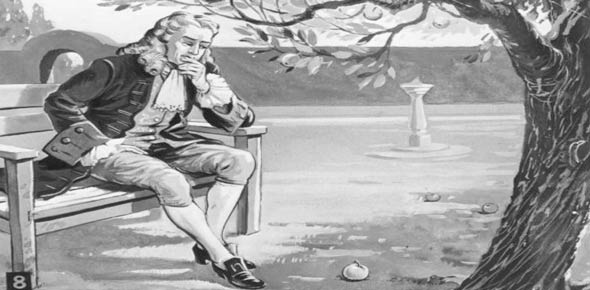# Newton's 2nd & 3rd Law

16 Questions | Attempts: 275
ShareSettingsNewton's 2nd + 3rd Laws

• 1.
A rock is propelled along a straight line in deep space by a constant force. If the force on the rocket decreases by half, its acceleration
• A.

Stays the same

• B.

Decreases by half

• C.

Triples

• D.

Doubles

• 2.
Two factors that greatly affect air resistance on falling objects are the
• A.

Surface area and speed

• B.

Surface texture and weight

• C.

Surface texture and mass

• D.

Surface area and gravity

• 3.
Terminal velocity occurs when
• A.

The net force on a falling object is zero

• B.

An object no longer accelerates during free fall

• C.

The air resistance on an object is equal to its weight

• D.

All of the above are correct

• 4.
A softball player hits a ball with a bat. The action force is the impact of the bat against the ball. The reaction this force is the
• A.

Weight of the ball

• B.

Air resistance of the ball

• C.

Force of the ball against the bat

• D.

The force of the grip of the player and the bat

• 5.
An unfortunate bug splatters on your windshield while you are driving your car. Compared to the force of the car on the bug, the force of the bug on the car is
• A.

Less and in the opposite direction

• B.

Greater and in the same direction

• C.

Equal and in opposite direction

• D.

Equal and in same direction

• 6.
In Question #5 above, does the bug or the car have the greater acceleration?
• A.

They both have the same acceleration

• B.

The car has the greater acceleration

• C.

The bug has the greater acceleration

• 7.
When a gun is fired, both the bullet and the gun undergo acceleration. The bullet's acceleration is
• A.

More than the gun's acceleration because it has less mass

• B.

More than the gun's acceleration because it receives more force

• C.

Less than the gun's acceleration because it receives less force

• D.

Less than the gun's acceleration because it has less mass

• 8.
Two people, one twice as massive as the other, attempt a tug-of-war with 12 meters of rope on frictionless ice. After a brief time they meet. The heavier person slides a distance of
• A.

4m

• B.

3m

• C.

5m

• D.

6m

• 9.
A pair of tennis balls fall through the air (with air resistance) from a tall building. One ball is regular and the other is filled with lead pellets. The ball to reach the ground first is the
• A.

Regular ball

• B.

• C.

Same

• 10.
A skydiver jumps from a high-flying plane. As her velocity of fall increases, her acceleration (remember the air resistance increases as the speed increases.)
• A.

Decreases

• B.

Increases

• C.

Unchanged

• 11.
A light woman and heavy man jump from an airplane at the same time and open their same-sized parachutes at the same time. Which person will get to a state of zero acceleration first?
• A.

Light woman

• B.

Both

• C.

Heavy man

• 12.
A block is dragged without acceleration in a straight line path across a level surface by a force 6N. what is the force of friction between the block and the surface?
• A.

Less than 6N

• B.

More than 6N

• C.

6N

• 13.
A push on a 1 kg brick accelerates the brick. Neglecting friction, to equally accelerate a 10kg brick, one would have to push with
• A.

1/10 the amount of force

• B.

100 times as much force

• C.

Just as much force

• D.

10 times as much force

• 14.
A girl pulls on a 10kg wagon with a constant force of 30N. What is the wagon's acceleration in meters per second per second?
• A.

300

• B.

10

• C.

30

• D.

3

• E.

.3

• 15.
If the acceleration of an object doubled, while the force applied to the object quadrupled, what must have happened to the mass of the object?
• A.

It doubled

• B.

It stayed the same

• C.

• D.

It was cut in half

• 16.
A 5kg block with an initial velocity of 10 m/s slides 10 m across a horizontal surface and comes to rest. It takes the block 2 seconds to stop. The stopping force acting on the block is about
• A.

25N

• B.

5N

• C.

10N

• D.

50N

## Related TopicsBack to top
×

Wait!
Here's an interesting quiz for you.